View markdown source on GitHub

# Feedforward neural networks (FNN) Deep Learning - Part 1

## Questions

• What is a feedforward neural network (FNN)?

• What are some applications of FNN?

## Objectives

• Understand the inspiration for neural networks

• Learn activation functions & various problems solved by neural networks

• Discuss various loss/cost functions and backpropagation algorithm

• Learn how to create a neural network using Galaxy’s deep learning tools

• Solve a sample regression problem via FNN in Galaxy

## Requirements

last_modification Last modification: Sep 30, 2023

# What is an artificial neural network?

Speaker Notes

What is an artificial neural network?

# Artificial Neural Networks

• ML discipline roughly inspired by how neurons in a human brain work
• Huge resurgence due to availability of data and computing capacity
• Various types of neural networks (Feedforward, Recurrent, Convolutional)
• FNN applied to classification, clustering, regression, and association

# Inspiration for neural networks

• Neuron a special biological cell with information processing ability
• Receives signals from other neurons through its dendrites
• If the signals received exceeds a threshold, the neuron fires
• Transmits signals to other neurons via its axon
• Synapse: contact between axon of a neuron and denderite of another
• Synapse either enhances/inhibits the signal that passes through it
• Learning occurs by changing the effectiveness of synapse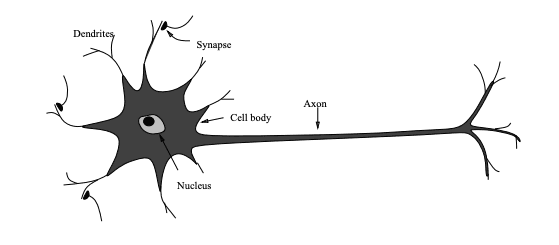# Celebral cortex

• Outter most layer of brain, 2 to 3 mm thick, surface area of 2,200 sq. cm
• Each neuron connected to 10^3 to 10^4 neurons
• Human brain has 10^14 to 10^15 connections

# Celebral cortex

• Neurons communicate by signals ms in duration
• Signal transmission frequency up to several hundred Hertz
• Millions of times slower than an electronic circuit
• Complex tasks like face recognition done within a few hundred ms
• Computation involved cannot take more than 100 serial steps
• The information sent from one neuron to another is very small
• Critical information not transmitted
• But captured by the interconnections
• Distributed computation/representation of the brain
• Allows slow computing elements to perform complex tasks quickly

# Perceptron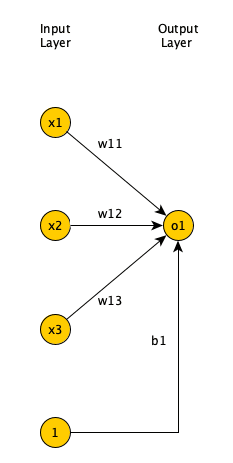# Learning in Perceptron

• Given a set of input-output pairs (called training set)
• Learning algorithm iteratively adjusts model parameters
• Weights and biases
• So the model can accurately map inputs to outputs
• Perceptron learning algorithm

# Limitations of Perceptron

• Single layer FNN cannot solve problems in which data is not linearly separable
• E.g., the XOR problem
• Adding one (or more) hidden layers enables FNN to represent any function
• Universal Approximation Theorem
• Perceptron learning algorithm could not extend to multi-layer FNN
• AI winter
• Backpropagation algorithm in 80’s enabled learning in multi-layer FNN

# Multi-layer FNN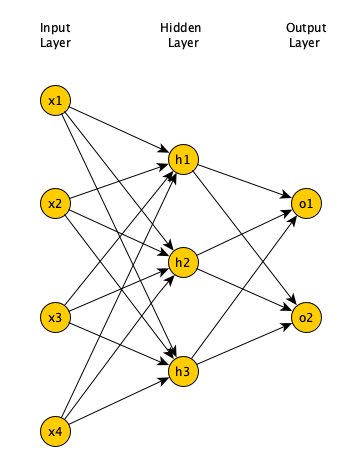• More hidden layers (and more neurons in each hidden layer)
• Can estimate more complex functions
• More parameters increases training time
• More likelihood of overfitting

# Activation functions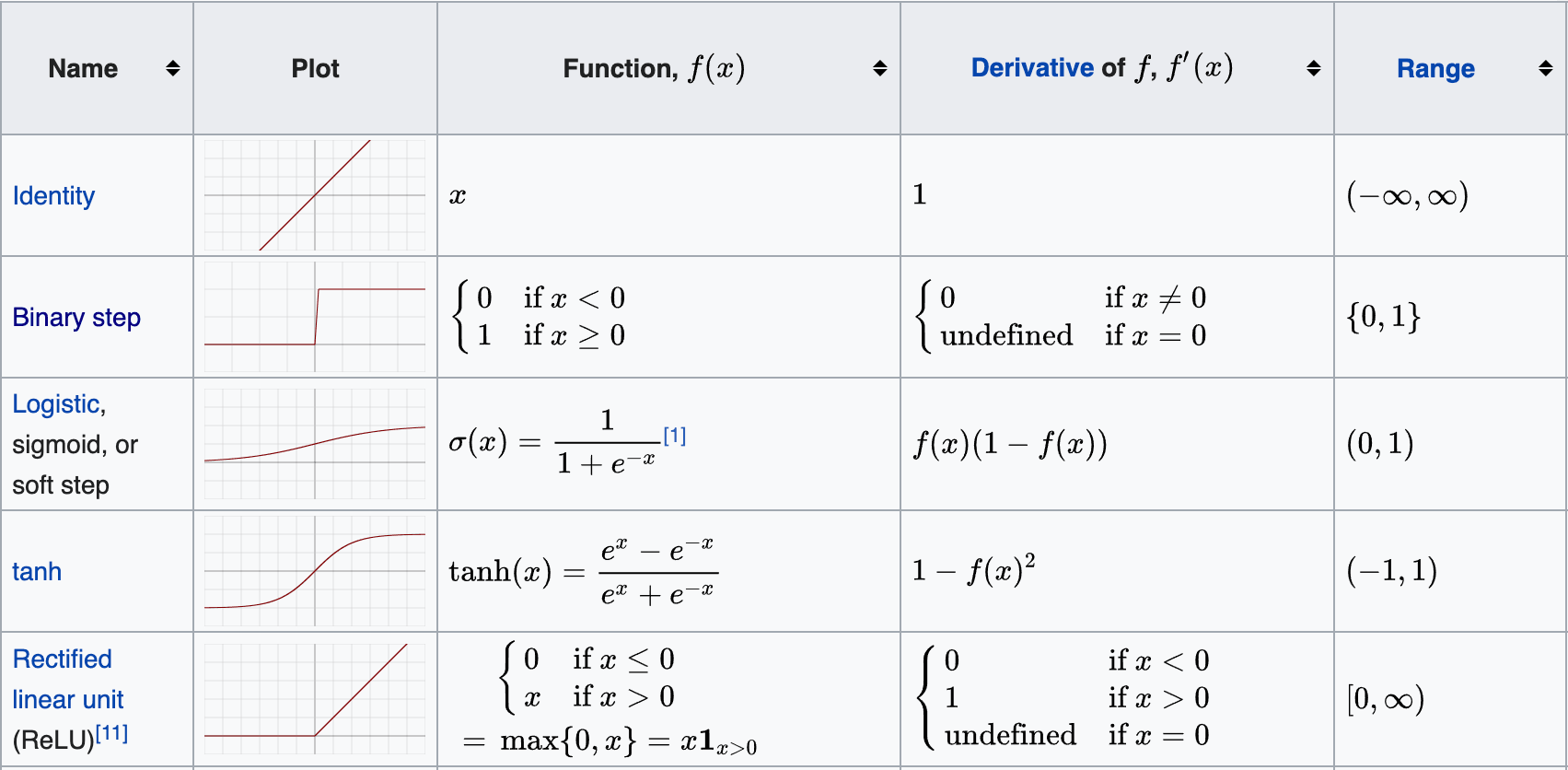# Supervised learning

• Training set of size m: { (x^1,y^1),(x^2,y^2),…,(x^m,y^m) }
• Each pair (x^i,y^i) is called a training example
• x^i is called feature vector
• Each element of feature vector is called a feature
• Each x^i corresponds to a label y^i
• We assume an unknown function y=f(x) maps feature vectors to labels
• The goal is to use the training set to learn or estimate f
• We want the estimate to be close to f(x) not only for training set
• But for training examples not in training set

# Classification problems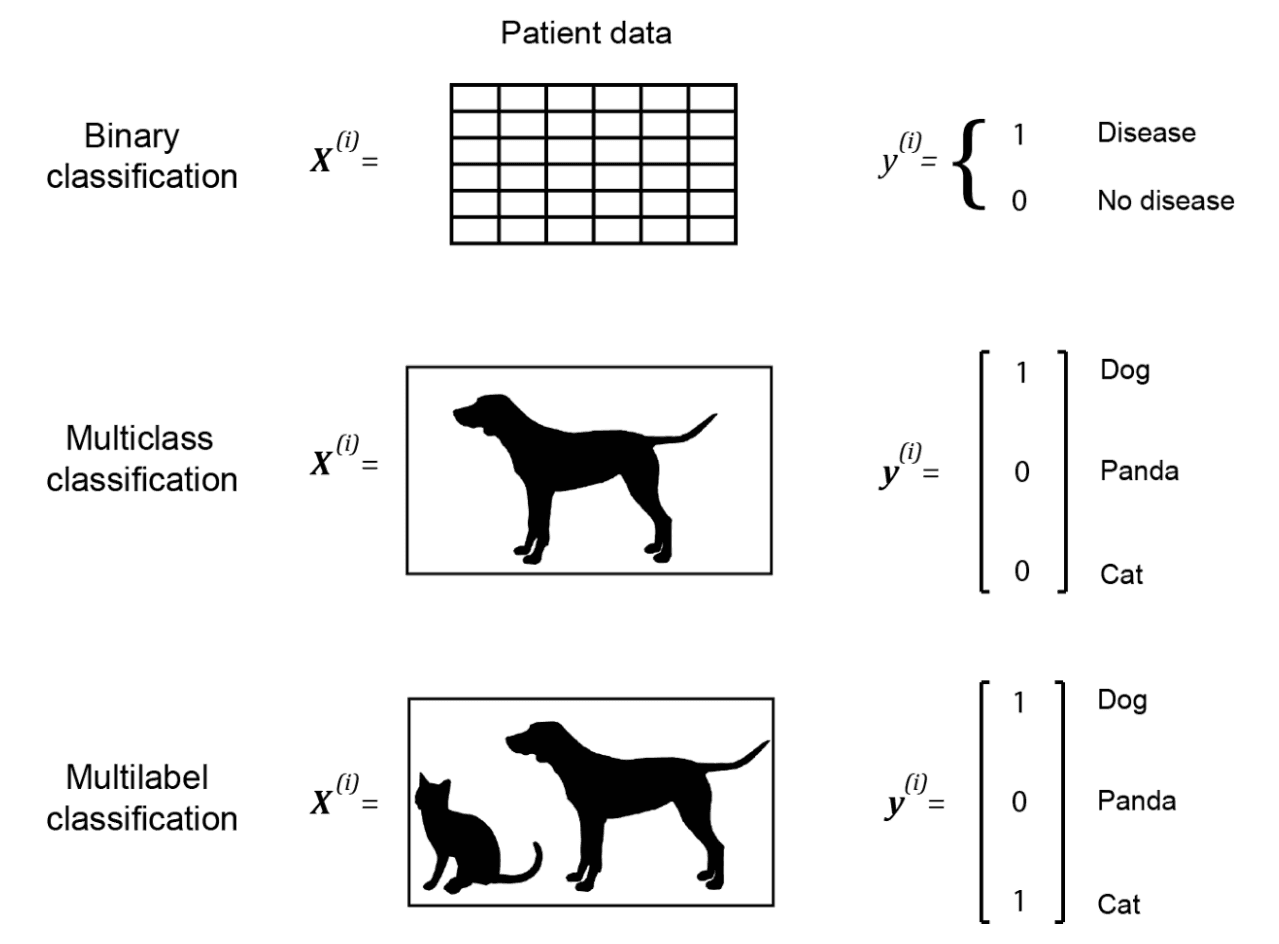# Output layer

• Binary classification
• Single neuron in output layer
• Sigmoid activation function
• Activation > 0.5, output 1
• Activation <= 0.5, output 0
• Multilabel classification
• As many neurons in output layer as number of classes
• Sigmoid activation function
• Activation > 0.5, output 1

# Output layer (Continued)

• Multiclass classification
• As many neurons in output layer as number of classes
• Softmax activation function
• Takes input to neurons in output layer
• Creates a probability distribution, sum of outputs adds up to 1
• The neuron with the highest proability is the predicted label
• Regression problem
• Single neuron in output layer
• Linear activation function

# Loss/Cost functions

• During training, for each training example (x^i,y^i), we present x^i to neural network
• Compare predicted output with label y^1
• Need loss function to measure difference between predicted & expected output
• Use Cross entropy loss function for classification problems
• And Quadratic loss function for regression problems
• Quadratic cost function is also called Mean Squared Error (MSE)

# Cross Entropy Loss/Cost functions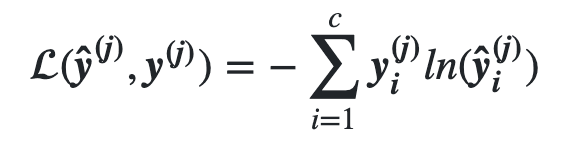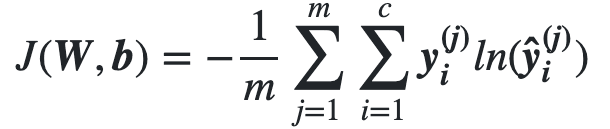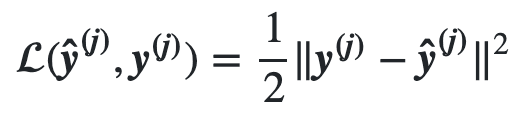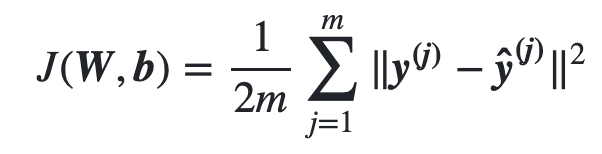# Backpropagation (BP) learning algorithm

• Find local minimum of a function by iteratively moving in opposite direction of gradient of function at current point
• Goal of learning is to minimize cost function given training set
• Cost function is a function of network weights & biases of all neurons in all layers
• Backpropagation iteratively computes gradient of cost function relative to each weight and bias
• Gradients (partial derivatives) are used to update weights and biases
• To find a local minimum

# Backpropagation error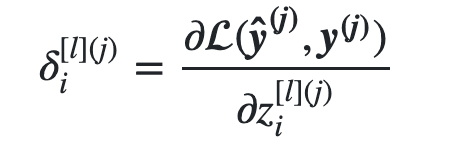# Backpropagation formulas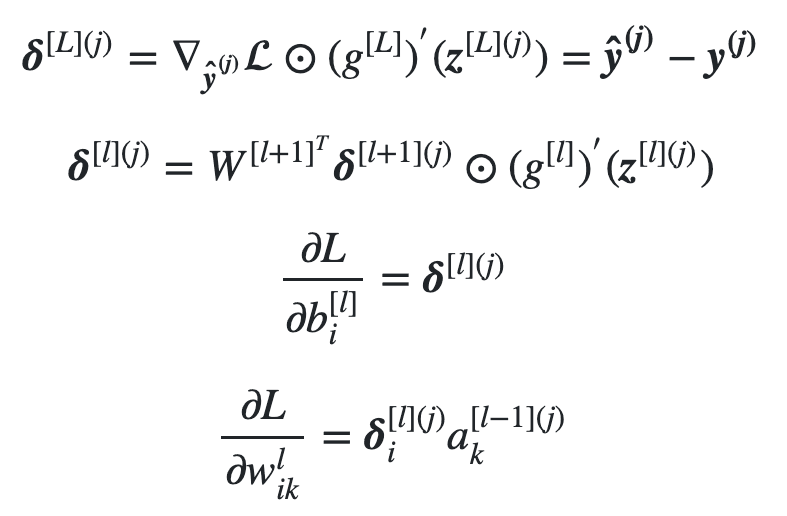• Calculate gradient for each weight/bias for all samples
• Average gradients and update weights/biases
• Slow, if we have too many samples
• Update weights/biases based on gradient of each sample
• Fast. Not accurate if sample gradient not representiative
• Middle ground solution
• Calculate gradient for each weight/bias for all samples in batch
• batch size is much smaller than training set size
• Average batch gradients and update weights/biases

• Second BP equation is recursive
• We have derivative of activation function
• Calc. error in layer prior to output: 1 mult. by derivative value
• Calc. error in two layers prior output: 2 mult. by derivative values
• If derivative values are small (e.g. for Sigmoid), product of multiple small values will be a very small value
• Since error values decide updates for biases/weights
• Update to biases/weights in first layers will be very small
• Slowing the learning algorithm to a halt
• The reason Sigmoid not used in deep networks
• Why ReLU is popular in deep networks

# Car purchase price prediction

• Given 5 features of an individual (age, gender, miles driven per day, personal debt, and monthly income)
• And, money they spent buying a car
• Learn a FNN to predict how much someone will spend buying a car
• We evaluate FNN on test dataset and plot graphs to assess the model’s performance
• Training dataset has 723 training examples
• Test dataset has 242 test examples
• Input features scaled to be in 0 to 1 range

# For references, please see tutorial’s References section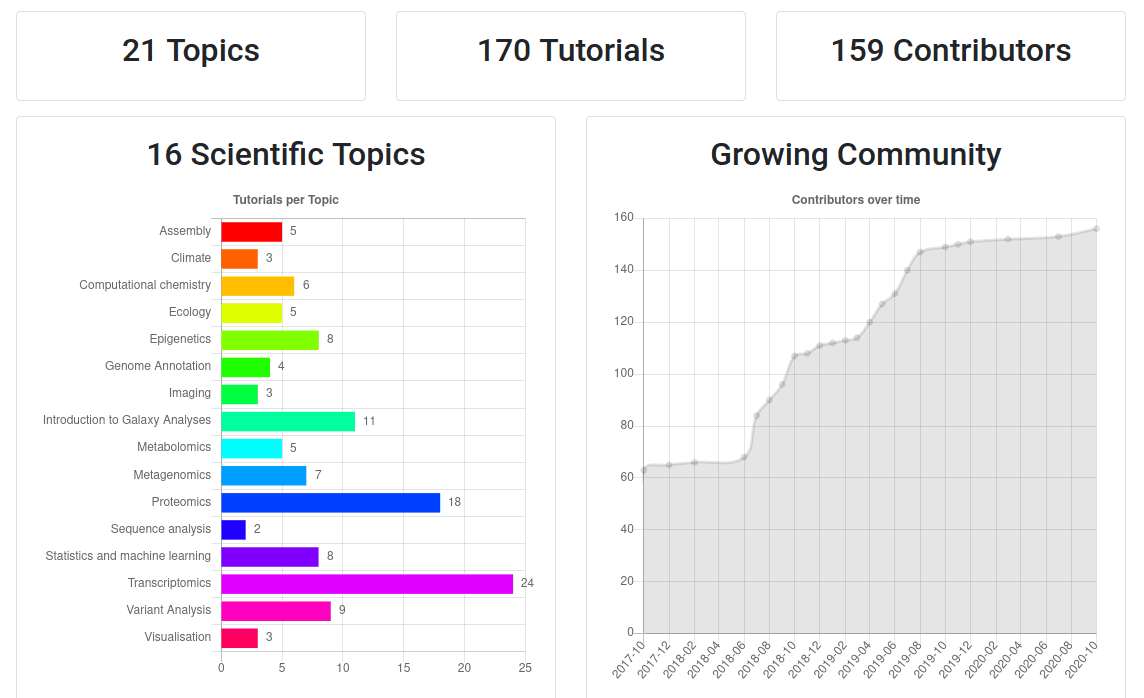Speaker Notes

• If you would like to learn more about Galaxy, there are a large number of tutorials available.
• These tutorials cover a wide range of scientific domains.

# Getting Help

Speaker Notes

• If you get stuck, there are ways to get help.
• Or you can chat with the community on Gitter.

# Join an event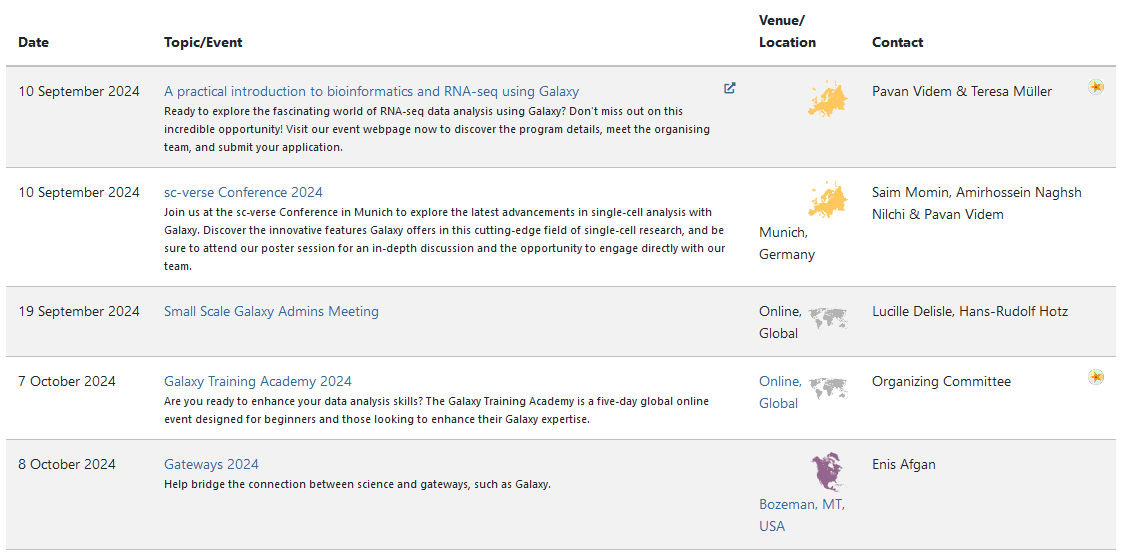Speaker Notes

• There are frequent Galaxy events all around the world.
• You can find upcoming events on the Galaxy Event Horizon.

## Thank you!

This material is the result of a collaborative work. Thanks to the Galaxy Training Network and all the contributors!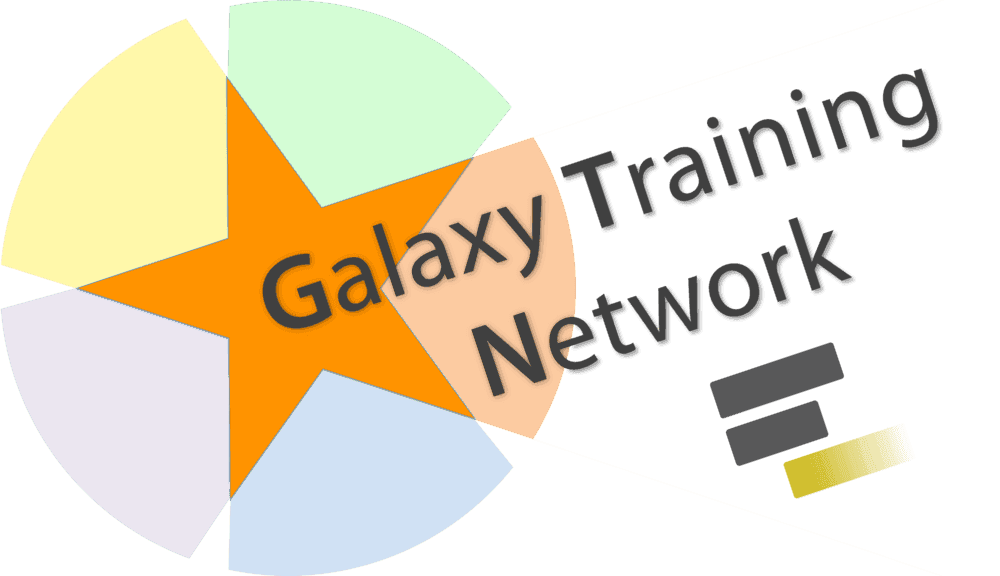This material is licensed under the Creative Commons Attribution 4.0 International License.